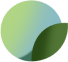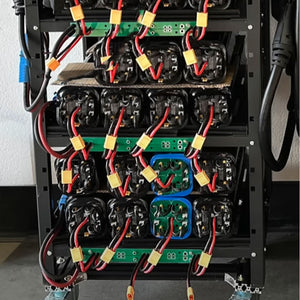All deliveries are carbon neutral# 4s2p, 10s3p? Understanding Battery Configurations

Many times while making battery purchases, you are bound to come up across terms defining different battery configurations and specs. This article makes an attempt to clearly detail these terms and help you make the right decisions while making these purchases. This is intended for beginner DIY enthusiasts and covers the basics and forms the building blocks to subsequent technical articles.

Series and Parallel Connections
To make a series connection, the negative terminal of one battery is connected to the positive terminal of the second battery. For a parallel connection, electrodes of same polarity are connected together, i.e., negative terminals are connected to the negative terminals, the same happens to the positive terminals too. Series connections increase the effective terminal voltage. If two batteries
with terminal voltages of 3V are connected in series, the effective voltage
is increased to 6V. For a series connection:The total voltage that a series circuit can supply is equal to the sum of all the individual voltage sources making up the circuit.
Parallel connections on the other hand increase the amp capacity on the other hand but the voltage remains the same despite having many cells forming the circuit. The reason for the increase in capacity is due to reduced internal resistances of the cell.

Example – An intuitive example is discussed here, using a BPS2 battery. This battery has a voltage of 24V and a capacity of 42.4Ah

Connecting two of these batteries in series will give; (24+24) V = 48 Volts
Connecting two of them in parallel will give; an increased amperage of (42.4+42.4) Ah = 84.8 Ah. The total voltage given out by these cells will however remain at 24 volts.

Combination of both Series and Parallel connection / Series-Parallel connections
To harness the benefits of both series and parallel connections, most battery configurations adopt both methods to increase output voltage of a battery at the same time the capacity of batteries. Mostly you will see them written depending on number of batteries used- there exist
different configurations.

Let’s take an example of a battery configuration with 7S2P
configuration. Still using the BPS2 Battery (24V, 42.4 Ah), if you were to make a 7S2P battery configuration of it

The series (S) part will give you a total voltage of (24*7) = 168 volts and the parallel part (P) a capacity of (42.4*2) = 84.8 Ah.
-
Battery Technical  Specifications
Just as the previous examples, let's use as an example a product that is available on the the JAG35.com website to get more understanding of these terms in real life situations. On this we are going to use an example the Boston swing 5300 custom-size 18650 cells:-
Ampere-hour (Ah) – This is the battery electric storage capacity; it specifies the amount of current a battery can discharge over a certain period of time. The table spec indicate that the Boston 5300 has an Ah rating of 5300mAh – this means that its capable of providing5300 milliamperes of current to a load for one hour or 2650 milliamperes for two hours.
-
Terminal Voltage/ Nominal Voltage (V) – The electrical potential at the output terminals of the battery.
-
Watt (W) – This gives the electrical power measure a battery is capable of providing. It’s the product of the nominal voltage and the output current.
-
Watt-hour (Wh) – This is the unit of electrical power or electrical energy given by a battery in one hour. In our study case, the nominal energy is 19.3Wh, this means that the battery is capable of giving 19.3 Watt in One hour.
-
Wh is a product of Ah and Voltage, Wh = Ah*V
-
On the load side, devices are also rated in watt-hours an electrical load rated for example let’s say 36Wh, needs 36 watts of power in an hour.
-
Wh is a product of Ah and Voltage.
-
Continuous discharge current (A) – The maximum amount of current the battery can provide to the load without overheating the battery.
-
Maximum charge rate (A) – The largest charge current that a battery can take in.
-
Nominal cell impedance - Also the cell internal resistance, the resistance within the battery itself – gives a magnitude of the opposition of the opposition of current flow in the battery. It is measured in Ohms. A battery’s internal resistance increases with temperature and use cycles.
-
Energy densities – There’s gravimetric and volumetric energy densities.
-
Gravimetric Energy Density – Gives a measure of available energy in watt – hours per unit mass of the battery i.e. Wh/kg.
-
Volumetric Energy density - Gives a measure of available energy in watt – hours per unit volume of the battery i.e. Wh/liter.
-
Knowledge of these terms are important in making the right purchase decisions for your DIY projects. Remember to share this with others via social media, text or email. Keep on building!